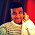## Induction

The natural numbers, denoted ${\Bbb N}$, are the counting numbers $\{ 0, 1, 2, 3, ... \}$.

If a statement about the natural numbers is true for some base case $n = n_0$ (usually $n_0 = 0$ or $n_0 = 1$), and if we can prove that if the statement is true for $n-1$, it is also true for $n$, then the statement is true for all natural numbers. This is the axiom of induction.

Basically, the axiom of induction is like dominoes- if the first domino falls, and if domino $(n-1)$'s falling knocks over domino $n$, then all the dominoes will fall.

This can be a powerful proof technique for statements about the natural numbers.

Here's an example:

Theorem: The sum of the natural numbers up to and including $n$, $\sum_{i=0}^{n}{i} = 0+1+2+...+n$, is equal to $\dfrac{n(n+1)}{2}$.

Proof: The base case where $n=1$ is true because $0+1 = 1 = \dfrac{2}{2} = \dfrac {(1)(2)}{2}$. For the induction step, assume (this assumption is called the induction hypothesis) that the statement is true for all natural numbers up to $n-1$. We need to prove it's true for $n$ as well.

Using the induction hypothesis after the second $=$ sign, we get: $$\sum_{i=0}^{n}{i} = \sum_{i=0}^{n-1}{i} + n = \dfrac{(n-1)(n)}{2} + n = \dfrac{(n-1)(n)}{2} + \dfrac{2n}{2} = \\[5mm] \dfrac{(n-1)(n)+2n}{2} = \dfrac{(n-1+2)(n)}{2} = \dfrac{n(n+1)}{2}$$ That proves the statement for $n$, so the theorem is proved for all natural numbers by the induction axiom.

1.The most easiest post of all, thus far:)

Question: Is proving by induction, fool-proof?

Base case: n=1
Only one horse in a set; So all horses in that set have same color.

Now assume n horses have same color;
Then set of n+1 horses will also have same color
exclude the last horse, n horses are of same color
exclude the 1st horse; still n horses are of same color

But this might contradict when n=2
If there are only 2 horses A & B, If we remove A, set has only B.. singleton set, so same color.
If we now remove horse B, only A remains; which "may or may not be" same color as of A.

So, Proof by Induction - Will it be valid? Or will lead to a Paradox?

2.Induction only works if the base case $n=n_0$ is correct and the induction step works for all $n \geq n_0$.

In the horse paradox, the base case $n=1$ is correct, but the induction step does not work when going from $n=1$ to $n=2$, because it assumes that the subsets $\{,2,..,n\}$ and $\{1,2,...,n-1\}$ have a common element, from which you conclude that the colors in the two subsets are the same. But when $n=2$, this is false, so the induction step doesn't work when going from 1 to 2.

So no, induction does not lead to paradoxes, but you need to be careful not to misuse it. Google induction pitfalls, and you'll find quite a few of them.